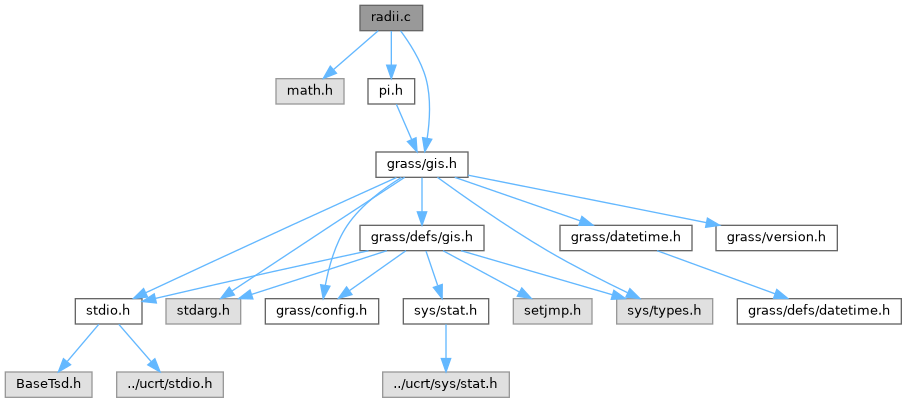GRASS GIS 8 Programmer's Manual  8.2.2dev(2023)-5c00b4e944

GIS Library - Calculating the Meridional Radius of Curvature. More...

#include <math.h>
#include <grass/gis.h>
#include "pi.h"Go to the source code of this file.

## Functions

double G_meridional_radius_of_curvature (double lon, double a, double e2)

double G_transverse_radius_of_curvature (double lon, double a, double e2)

double G_radius_of_conformal_tangent_sphere (double lon, double a, double e2)
Radius of conformal tangent sphere. More...

## Detailed Description

GIS Library - Calculating the Meridional Radius of Curvature.

Todo:

Comments: on page http://www.mentorsoftwareinc.com/cc/gistips/TIPS0899.HTM down where it says "Meridional Radius of Curvature" is the exact formula out of "radii.c". Quote: "essentially, the radius of curvature, at a specific latitude ...".

See also http://williams.best.vwh.net/ellipsoid/node1.html which has a nice picture showning the parametric latitude and phi, the geodetic latitude. On the next page, http://williams.best.vwh.net/ellipsoid/node2.html, in equation 3, the Meridional Radius of Curvature shows up.

So, it looks like you are calculating the Meridional Radius of Curvature as a function of GEODETIC LATITUDE.

Various formulas for the ellipsoid. Reference: Map Projections by Peter Richardus and Ron K. Alder University of Illinois Library Call Number: 526.8 R39m Parameters are:

• lon = longitude of the meridian
• a = ellipsoid semi-major axis
• e2 = ellipsoid eccentricity squared

meridional radius of curvature (p. 16)

                       2
a ( 1 - e )
M = ------------------
2   2    3/2
(1 - e sin lon)

transverse radius of curvature (p. 16)

                   a
N = ------------------
2   2    1/2
(1 - e sin lon)

radius of the tangent sphere onto which angles are mapped conformally (p. 24)

      R = sqrt ( N * M )

(C) 2001-2009 by the GRASS Development Team

This program is free software under the GNU General Public License (>=v2). Read the file COPYING that comes with GRASS for details.

## Function Documentation

 double G_meridional_radius_of_curvature ( double lon, double a, double e2 )

Returns the meridional radius of curvature at a given longitude:Parameters
 lon longitude a ellipsoid semi-major axis e2 ellipsoid eccentricity squared
Returns

Definition at line 80 of file radii.c.

 double G_radius_of_conformal_tangent_sphere ( double lon, double a, double e2 )

Returns the radius of the conformal sphere tangent to ellipsoid at a given longitude:Parameters
 lon longitude a ellipsoid semi-major axis e2 ellipsoid eccentricity squared
Returns

Definition at line 133 of file radii.c.

 double G_transverse_radius_of_curvature ( double lon, double a, double e2 )